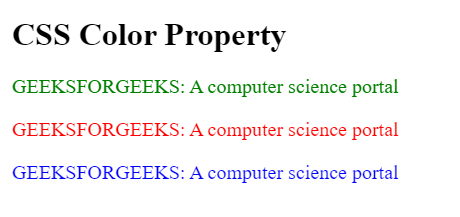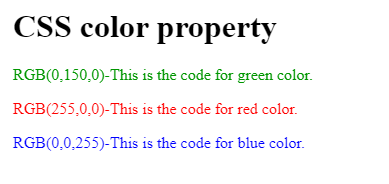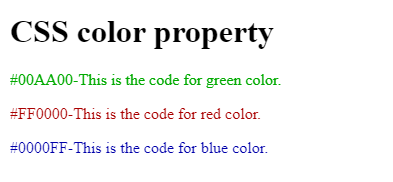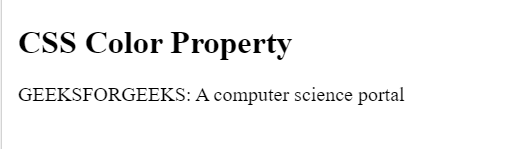# CSS color Property

The color property in CSS is used to set the color of text, the background of the webpage, and also to set the color of borders.

Syntax

`color: color/initial/inherit;`

Property Values:

1. color: It will set the color to the text which the programmer specifies in the CSS file. The color can be set to the text in 4 forms-

2. color-name: By directly specifying the name of the color like blue, green, yellow, white, black, etc.

Syntax:

`color: name-of-the-color; `

Example:

## HTML

 `` `<``html``>` `<``head``>` `    ``<``title``>` `        ``CSS color-name property` `    ``` `    ``//Below is an example of Internal CSS` `    ``<``style``>` `        ``h1 {` `            ``color: black;` `        ``}` `        ``p {` `            ``font-size: 20px;` `            ``color: green;` `        ``}` `        ``.gfg1 {` `            ``font-size: 20px;` `            ``color: red;` `        ``}` `        ``.gfg2 {` `            ``font-size: 20px;` `            ``color: blue;` `        ``}` `    ``` ``   `<``body``>` `    ``<``h1``>` `        ``CSS Color Property` `    ```   `    ``<``p``>` `        ``GEEKSFORGEEKS: A computer science portal` `    ```   `    ``<``p` `class``=``"gfg1"``>` `        ``GEEKSFORGEEKS: A computer science portal` `    ```   `    ``<``p` `class``=``"gfg2"``>` `        ``GEEKSFORGEEKS: A computer science portal` `    ``` `` ``

Output:3. RGB/RGBA Value: Here R stands for Red, G stands for Green, and B stands for Blue. The color will be assigned to the text by using the range of these values. These values range from 0 to 255. And, A stands for Alpha channel. Which represents the opacity or opaque of the color.

Syntax:

`color: RGBA(value, value, value, value);`

Example:

## HTML

 `` `<``html``>` `<``head``>` `    ``<``title``>` `        ``CSS RGB value property` `    ``` `    ``<``style``>` `        ``h1 {` `            ``color: RGB(0, 0, 0);` `        ``}` `        ``p {` `            ``color: RGB(0, 150, 0);` `        ``}` `        ``.gfg1 {` `            ``color: RGB(255, 0, 0);` `        ``}` `        ``.gfg2 {` `            ``color: RGB(0, 0, 255);` `        ``}` `    ``` ``   `<``body``>` `    ``<``h1``>` `        ``CSS color property` `    ```   `    ``<``p``>` `        ``RGB(0, 150, 0)-This is the code for green color.` `    ```   `    ``<``p` `class``=``"gfg1"``>` `        ``RGB(255, 0, 0)-This is the code for red color.` `    ```   `    ``<``p` `class``=``"gfg2"``>` `        ``RGB(0, 0, 255)-This is the code for blue color.` `    ``` `` ``

Output:4. Hexa-Decimal Value: It represents the value of the color in hexadecimal format. It should start with the prefix #. These values range from #000000 to #FFFFFF. And, If there is an alpha channel that defines the opacity of the color, then we will represent it by adding FF (if 100%) after the hex code.

Syntax:

`color: #RRGGBBFF;`

Example:

## HTML

 `` `<``html``>` `<``head``>` `    ``<``title``>` `        ``CSS Hexa-decimal value property` `    ``` `    ``<``style``>` `        ``body {` `            ``background-color: rgb(200, 200, 200);` `        ``}` `        ``h1 {` `            ``color: #000000;` `        ``}` `        ``p {` `            ``color: #00aa00;` `        ``}` `        ``.gfg1 {` `            ``color: #ff0000;` `        ``}` `        ``.gfg2 {` `            ``color: #0000ff;` `        ``}` `    ``` ``   `<``body``>` `    ``<``h1``>` `        ``CSS color property` `    ```   `    ``<``p``>` `        ``#00AA00-This is the code for green color.` `    ```   `    ``<``p` `class``=``"gfg1"``>` `        ``#FF0000-This is the code for red color.` `    ```   `    ``<``p` `class``=``"gfg2"``>` `        ``#0000FF-This is the code for blue color.` `    ``` `` ``

Output:5. HSL/HSLA values: HSL stands for Hue, Saturation, and Lightness. The range of hue will be from (0 to 360 degree), saturation means the Grey effect it ranges from (0 to 100%), and Lightness means the effect of light which ranges from (0 to 100%).

Syntax:

`color: HSL(value, value, value);`

Example:

## HTML

 `` `<``html``>`   `<``head``>` `    ``<``title``>` `        ``CSS HSL value property` `    ``` `    ``<``style``>` `        ``body {` `            ``background-color: white;` `        ``}` `        ``h1 {` `            ``color: HSL(0, 0, 0);` `        ``}` `        ``p {` `            ``color: HSL(147, 50%, 47%);` `        ``}` `        ``.gfg1 {` `            ``color: HSL(0, 100%, 50%);` `        ``}` `        ``.gfg2 {` `            ``color: HSL(240, 100%, 50%);` `        ``}` `    ``` ``   `<``body``>` `    ``<``h1``>` `        ``CSS Color property` `    ```   `    ``<``p``>` `        ``HSL(147, 50%, 47%)-This is the code for green color.` `    ```   `    ``<``p` `class``=``"gfg1"``>` `        ``HSL(0, 100%, 50%)-This is the code for red color.` `    ```   `    ``<``p` `class``=``"gfg2"``>` `        ``HSL(240, 100%, 50%)-This is the code for blue color.` `    ```   `` ``

Output:6. initial: This value will set the value of the color to its default value.

Syntax:

`color: initial;`

Example:

## HTML

 `` `<``html``>` `<``head``>` `    ``<``title``>` `        ``CSS color-name property` `    ``` `    ``` `    ``<``style``>` `        ``h1 {` `            ``color: black;` `        ``}` `        ``p {` `            ``font-size: 20px;` `            ``color: initial;` `        ``}` `    ``` ``   `<``body``>` `    ``<``h1``>` `        ``CSS Color Property` `    ```   `    ``<``p``>` `        ``GEEKSFORGEEKS: A computer science portal` `    ``` `` ``

Output:7. inherit: It will inherit the property of the color from its parent element.

Supported browsers: The browsers supported by the color property are-